Cross Reference Down

Kozlov, VV (2005). Weighted averages, uniform distribution, and strict ergodicity. Russian Mathematical Surveys 60(6), pp. 1121-1146.

This work cites the following items of the Benford Online Bibliography:

 Benford, F (1938). The law of anomalous numbers. Proceedings of the American Philosophical Society, Vol. 78, No. 4 (Mar. 31, 1938), pp. 551-572.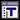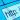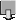Cigler, J (1964). Methods of summability and uniform distribution mod 1. Compositio Mathematica 16, pp. 44-51.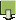Diaconis, P (1977). The Distribution of Leading Digits and Uniform Distribution Mod 1. Annals of Probability 5(1), pp. 72-81. ISSN/ISBN:0091-1798.Duncan, RL (1969). Note on the initial digit problem. Fibonacci Quarterly 7(5), pp. 474-475.Feller, W (1971). An Introduction to Probability Theory and Its Applications. 2nd ed., J. Wiley (see p 63, vol 2).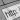Flehinger, BJ (1966). On the Probability that a Random Integer has Initial Digit A. American Mathematical Monthly 73(10), pp. 1056-1061. ISSN/ISBN:0002-9890. DOI:10.2307/2314636.Kuipers, L and Niederreiter, H (1974). Uniform Distribution of Sequences. J. Wiley; newer edition - 2006 from Dover. ISSN/ISBN:0486450198.Newcomb, S (1881). Note on the frequency of use of the different digits in natural numbers. American Journal of Mathematics 4(1), pp. 39-40. ISSN/ISBN:0002-9327. DOI:10.2307/2369148.Raimi, RA (1976). The First Digit Problem. American Mathematical Monthly 83(7), pp. 521-538. ISSN/ISBN:0002-9890. DOI:10.2307/2319349.Tsuji, M (1952). On the uniform distribution of numbers mod 1. Journal of the Mathematical Society of Japan 4(3/4), pp. 313-322. DOI:10.2969/jmsj/00430313.